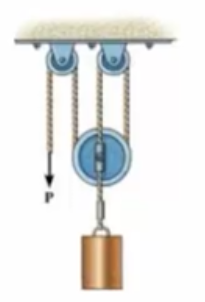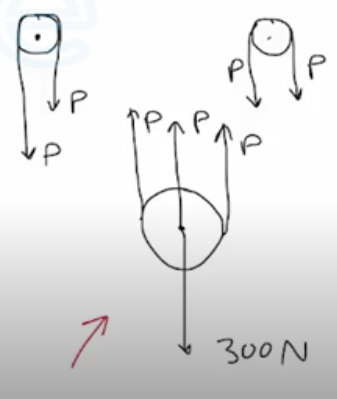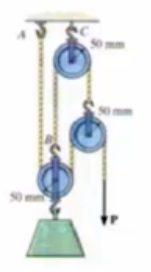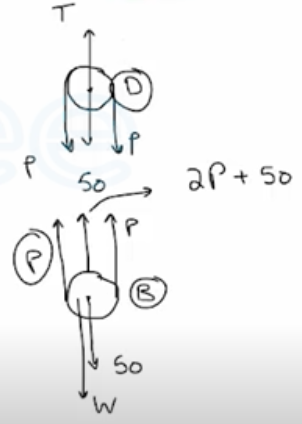Need Help?

Subscribe to Statics

###### \${selected_topic_name}
• Notes

Determine the force  $P$  needed to hold the  $300-N$  weight in equilibrium.$+\uparrow \sum F_ y=0$

$3 P-300=0$

$\rightarrow \quad 3 P=300$

$∴ P=100 N$

Determine the force  $\mathbf{P}$  needed to support the  $50-kg$  weight. Each pulley has a weight of  $50 N$  . Also, what are the cord reactions at  $A$  and  $B$ ?$w=50 * 9.81=490.5 N$

D

$\uparrow \quad \sum F_{y}=0 \quad \longrightarrow T-P-P-50=0$

$T=2 P+50$

B

$+\uparrow \sum F_ y=0$

$P+P+(2P+50)-W-50=0$

$4 P=490.5 \longrightarrow P=122.6 N$

$∴ T_{A}=P=122.6 N$

$T_{B}=2 P+50=2(122 \cdot 6)+50=295.25 N$